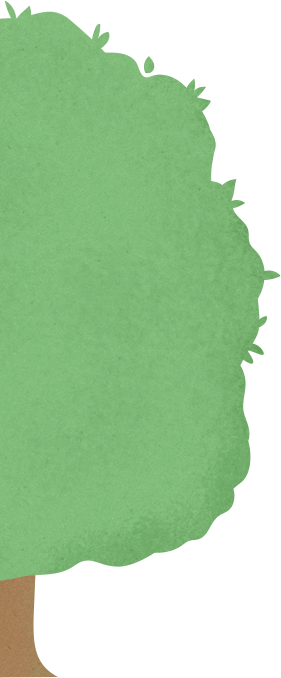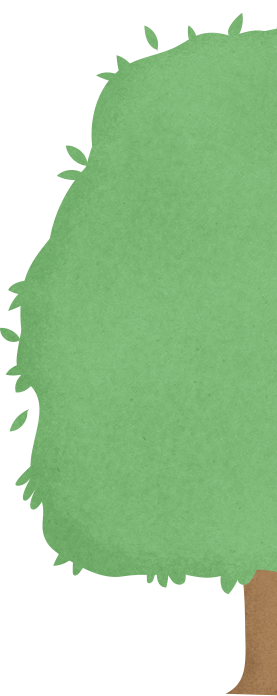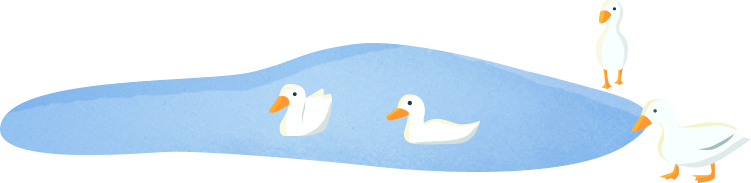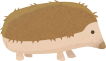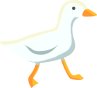## Maths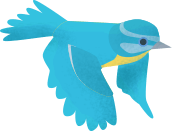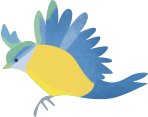# Maths

This week we are thinking about: half and quarters

Please use the maths hub resources/sheets this week. These can be found in the Year 1 section of the school website.

Underneath for each day will be other activities that can be done.

Day 1

Find half of objects

Take some fruit/veg/paper, practise cutting in half. making the object into 2 equal pieces.

Discuss that it is only in half if the two pieces are equal/the same.

Fold 2d shapes in half e.g cut out a square/circle/rectangle. Colour in half of the shape.

Maths hub sheet 1

Day 2

Find half of amounts

Count out differencing amounts of objects depending on child’s ability. Make sure they are numbers that can be divided by 2. Split these fairly into 2 groups. E.g half of 14 = 7

Maths hub sheet 2

Day 3

Find quarters of objects

Take some fruit/veg/paper, practise cutting in quarters. making the object into 4 equal pieces.

Discuss that it is only in quarters if the four pieces are equal/the same.

Fold 2d shapes in quarters e.g cut out a square/circle/rectangle. Colour in one quarter of the shape.

Maths hub sheet 3

Day 4

Find quarters of amounts

Count out differencing amounts of objects depending on child’s ability. Make sure they are numbers that can be divided by 4. Split these fairly into 4 groups. E.g one quarter of 12 = 3

Maths hub sheet 4

Day 5

Can you find one half and one quarter of all these numbers?

12          20           16           18            28           32

Which number does not divide by both 2 and 4?